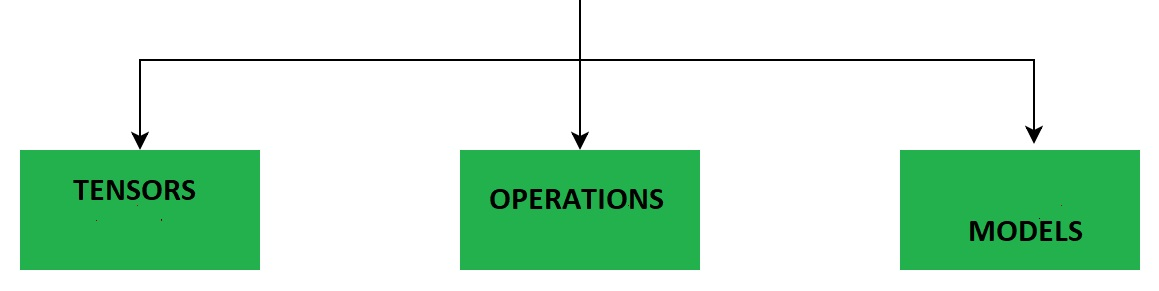# TensorFlow Models

## TesorFlow.js

A JavaScript Library for

### Training and DeployingMachine Learning ModelsIn the Browser## Tensorflow Models

Models and Layers are important building blocks in Machine Learning.

For different Machine Learning tasks you must combine different types of Layers into a Model that can be trained with data to predict future values.

TensorFlow.js is supporting different types of Models and different types of Layers.

A TensorFlow Model is a Neural Network with one or more Layers.

## A Tensorflow Project

A Tensorflow project has this typical workflow:

• Collecting Data
• Creating a Model
• Adding Layers to the Model
• Compiling the Model
• Training the Model
• Using the Model

## Example

Suppose you knew a function that defined a strait line:

Y = 1.2X + 5

Then you could calculate any y value with the JavaScript formula:

y = 1.2 * x + 5;

To demonstrate Tensorflow.js, we could train a Tensorflow.js model to predict Y values based on X inputs.

The TensorFlow model does not know the function.

// Create Training Data
const xs = tf.tensor([0, 1, 2, 3, 4]);

// Define a Linear Regression Model
const model = tf.sequential();

// Specify Loss and Optimizer
model.compile({loss:'meanSquaredError', optimizer:'sgd'});

// Train the Model
model.fit(xs, ys, {epochs:500}).then(() => {myFunction()});

// Use the Model
function myFunction() {
const xArr = [];
const yArr = [];
for (let x = 0; x <= 10; x++) {
xArr.push(x);
let result = model.predict(tf.tensor([Number(x)]));
result.data().then(y => {
yArr.push(Number(y));
if (x == 10) {plot(xArr, yArr)};
});
}
}

Try it Yourself »

## Collecting Data

Create a tensor (xs) with 5 x values:

const xs = tf.tensor([0, 1, 2, 3, 4]);

Create a tensor (ys) with 5 correct y answers (multiply xs with 1.2 and add 5):

## Creating a Model

Create a sequential mode:.

const model = tf.sequential();

In a sequential model, the output from one layer is the input to the next layer.

Add one dense layer to the model.

The layer is only one unit (tensor) and the shape is 1 (one dimentional):

in a dense the layer, every node is connected to every node in the preceding layer.

## Compiling the Model

Compile the model using meanSquaredError as loss function and sgd (stochastic gradient descent) as optimizer function:

model.compile({loss:'meanSquaredError', optimizer:'sgd'});

## Tensorflow Optimizers

• Ftrl - Implements the FTRL algorithm.
• Optimizer - Base class for Keras optimizers.
• RMSprop - Implements the RMSprop algorithm.
• SGD - Stochastic Gradient Descent Optimizer.

## Training the Model

Train the model (using xs and ys) with 500 repeats (epochs):

model.fit(xs, ys, {epochs:500}).then(() => {myFunction()});

## Using the Model

After the model is trained, you can use it for many different purposes.

This example predicts 10 y values, given 10 x values, and calls a function to plot the predictions in a graph:

function myFunction() {
const xArr = [];
const yArr = [];
for (let x = 0; x <= 10; x++) {
let result = model.predict(tf.tensor([Number(x)]));
result.data().then(y => {
xArr.push(x);
yArr.push(Number(y));
if (x == 10) {display(xArr, yArr)};
});
}
}

Try it Yourself »

This example predicts 10 y values, given 10 x values, and calls a function to display the values:

function myFunction() {
const xArr = [];
const yArr = [];
for (let x = 0; x <= 10; x++) {
let result = model.predict(tf.tensor([Number(x)]));
result.data().then(y => {
xArr.push(x);
yArr.push(Number(y));
if (x == 10) {display(xArr, yArr)};
});
}
}

Try it Yourself »

W3Schools is optimized for learning and training. Examples might be simplified to improve reading and learning. Tutorials, references, and examples are constantly reviewed to avoid errors, but we cannot warrant full correctness of all content. While using W3Schools, you agree to have read and accepted our terms of use, cookie and privacy policy.